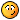# averages for cells

J

#### jatman

i have a spreadsheet that calculates averages, but as i add more columns, it
always calculates the same columns:

B F G H I J
ITEM AVG # ## # #

the formula that i am using is =(SUM(G3:O3)-MIN(G3:O3)-MAX(G3:O3))/7 (drops
the lowest and highest and make an olympic average to use).

when i insert another row of the most recent data on the left side (push old
data to the right, but keep the information). i insert a column between f
and g (new column is now g...). the formula changes and continues to use the
same values and not the new values...

formula changes to =sum(h33)-min(h33)-max(h33))/7

how do i get it the formula to limit itself to just the original selection,
even if i insert columns in the selection?

thank you,

jat

G

#### Glenn

jatman said:
i have a spreadsheet that calculates averages, but as i add more columns, it
always calculates the same columns:

B F G H I J
ITEM AVG # ## # #

the formula that i am using is =(SUM(G3:O3)-MIN(G3:O3)-MAX(G3:O3))/7 (drops
the lowest and highest and make an olympic average to use).

when i insert another row of the most recent data on the left side (push old
data to the right, but keep the information). i insert a column between f
and g (new column is now g...). the formula changes and continues to use the
same values and not the new values...

formula changes to =sum(h33)-min(h33)-max(h33))/7

how do i get it the formula to limit itself to just the original selection,
even if i insert columns in the selection?

thank you,

jat

=(SUM(INDIRECT("G3:O3"))-MIN(INDIRECT("G3:O3"))-MAX(INDIRECT("G3:O3")))/7

F

#### FSt1

hi
you need to understand the differenct between relative reference and
absolute reference. look that up in xl help
i think in your situation you want absolute reference or reference that
don't change.
relative references change when you move them or move the formula.
to change form relatitive to absolute references and dollar signs i.e.
=(SUM(\$G\$3:\$O\$3)-MIN(\$G\$3:\$O\$3)-MAX(\$G\$3:\$O\$3))/7

you can put absolute reference on column, row, both. xl help will explain
all that.
regards
FSt1

K

#### klswvu

(SUM(OFFSET(F3,0,1,1,9))-MIN(OFFSET(F3,0,1,1,9))-MAX(OFFSET(F3,0,1,1,9)))/7

Third Option... Offset

G

#### Glenn

Put your formula in A1 and then insert a column at G (per the OP's
instructions). Now what's in A1?

J

#### jatman

Column A has data (category for items in column B).

jat

G

#### Glenn

My response was for FSt1, whose recommendation didn't work. Please see my other
response for something that will work.

Sorry for the confusion.